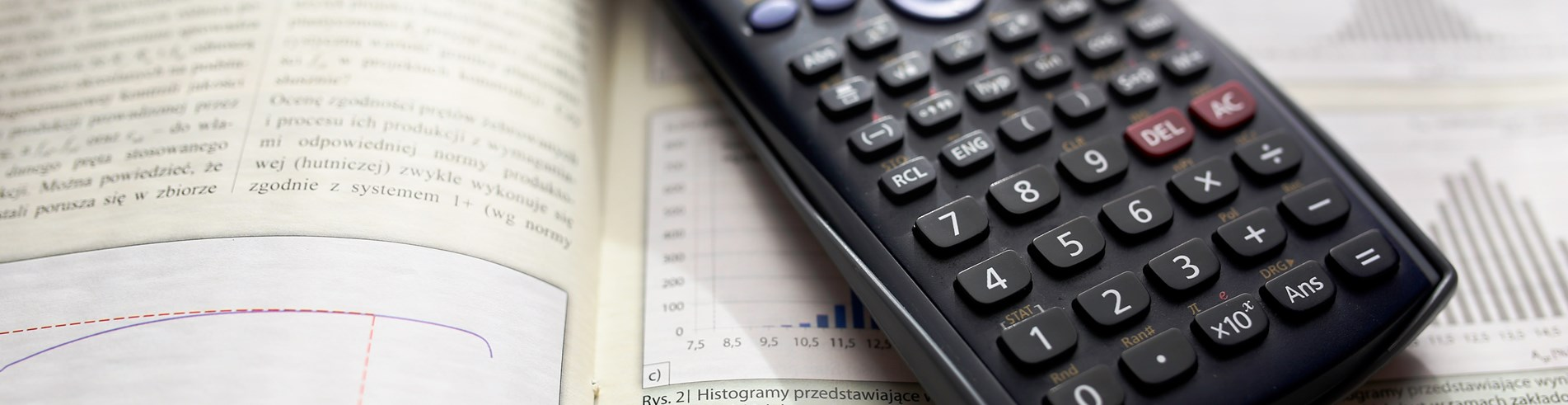Close# Maths

Mathematics is a core subject and as such is studied by every student in the school from Year 7 to Year 11. Maths is an all embracing and pervading part of life, helping us to create, plan, organise and solve problems.

Mathematics is the universal language of our environment, helping mankind explain and create within it for thousands of years. From playing games to playing music, maths is vital to helping students fine tune their creativity and turn their dreams into reality.

Our curriculum is based on the national programmes of study and lessons are accessible to all students in the class to create opportunities and build familiarity and accuracy in techniques and allow students to use their skills in everyday life. We cover a variety of topics that allow students to excel in their learning in different fields. Students know that when they come to lessons, they will be entering a positive environment for learning where they learn new techniques and challenges are accepted.

We revisit topics throughout KS3 and 4 to consolidate understanding and encourage students to link their work with other areas to extend their thinking. We have a strong focus on developing problem-solving skills alongside mathematical content to ensure we develop resilient, independent learners ready to tackle GCSE and A-level maths.

The department offers some small group interventions during the school year, these are designed to help students conquer any issues with topics that have arisen during lessons as well as preparing students for external examinations.

The department runs a board games club that encourages students to develop their use of strategy and problem solving. This club promotes fairness within a competitive environment.

##### Curriculum Information

 Year Autumn Spring Summer 7 Number and calculations Factors and multiples Area and volume Fractions  Expressions Fractions, decimals and percentages Charts and averages Shapes and angles Ratio Equations Equations  Sequences Graphs 8 Number Area and volume Expressions Fractions, decimals and percentages Probability Equations Shapes and angles Ratio Pythagoras and trigonometry Graphs Sequences Charts and averages Transformations 9 Factors and multiples Indices and standard form Expressions and equations Charts and averages Area and volume Fractions, decimals and percentages Ratio Shapes and angles Pythagoras’ theorem Right angled Trigonometry Probability 10 Indices and standard form Linear and quadratic expressions and equations Charts and averages  Cumulative frequency Transformations Area and volume Fractions, decimals and percentages  Linear graphs Constructions Simultaneous equations Ratio and proportion Shape and angles Circle theorems Graphs of other functions  Pythagoras’ theorem Right angled and non-right-angled trigonometry 11 Non-right-angled trigonometry Algebraic manipulation  Quadratic equations   Functions  Algebraic proof Vectors Surds  Ratio and proportion  Linear and quadratic graphs Cumulative frequency Circle theorems Linear and quadratic simultaneous equations

I enjoy maths because I can see how topics are linked together
Year 9 student
The regular exam practice we do has help me focus on a small range of topics, the feedback and follow up has helped in improving my grade
Year 11 student